## Upcoming Assignments/Quizzes

Assignments Open Time Due Time
Data Visualization Friday, Sept 14 (1:00 am) Sunday, Sept 23 (11:55 pm EST)
Module 4 Data Quiz Friday, Sept 21 (1:00 am EST) Sunday, Sept 23 (11:55 pm EST)
Module 4 Conceptual Quiz Friday, Sept 21 (1:00 am EST) Sunday, Sept 23 (11:55 pm EST)

## Notes from Discussion Board/Office Hours

### Fixed-width files

In one of the lectures and data exercises this week we used a fixed-width file (FWF). This file type can be useful for computing with very large files. Unlike CSV files, FWF structure columns by defining the exact number of characters in each column, spaces are used to fill out the remaining character spaces. This is why we need to define the widths when we use the read.fwf() function.

### LOWESS

In this module, we also use a function called lowess() to draw a fitted line to express trends in out data. This function fits a LOWESS (or LOESS) model, which stands for Locally Weighed Smoothing. This is an example of a non-parameteric approach for modelling data, which means that we don’t make any assumptions about the shape or distribution of the data. This is different than a parametric model, like a linear regression, where we explicitly state the shape of the function in our model we’re using to fitting with the data.

LOWESS uses a group of nearby by points to construct the trend line, which is really useful if the shape of the trend cannot be easily defined. Here’s an example of the difference between a LOWESS model (in red) and a linear model (in blue) using some fake (or generative) data:

# Create fake data
x <- runif(100, -1, 1) # 100 random samples between -1 and 1
y <- x^3 + 2*x^2 + rnorm(length(x), 0, 0.1) # the rnorm part adds some error

# Make models for the trend lines
linear <- lm(y ~ x)
lowess <- lowess(x,y)

# Plot
plot(x,y)
abline(linear, col = "blue", lwd = 2)
lines(lowess$x, lowess$y, col = "red", lwd = 2)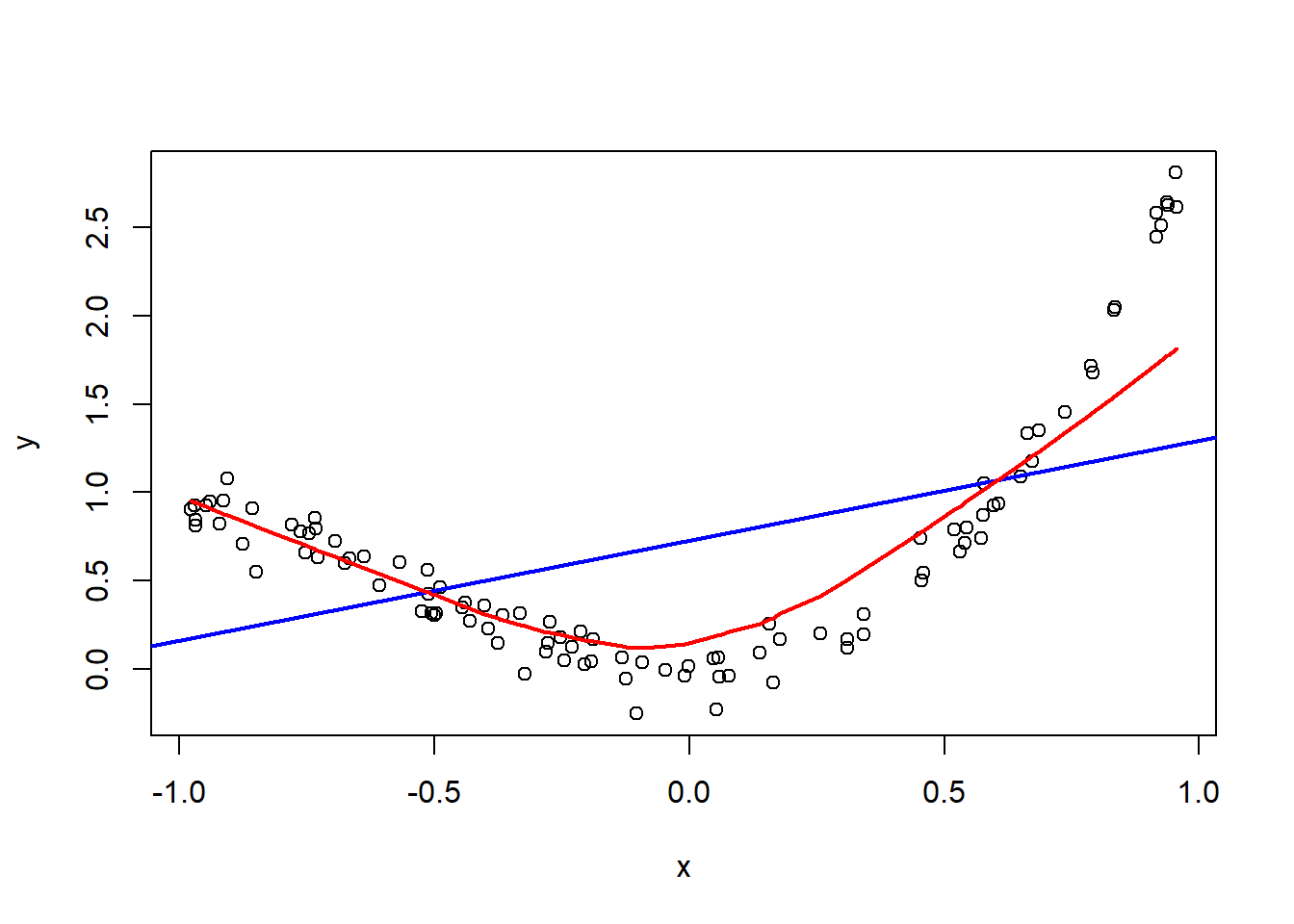### Using ~ versus , in the plot() and lowess() functions

Recall from last module that in R the ~ (called a tilde) is an operator used to define a formula. You can think of this as reading as “as a function of” or “depends on”, so y ~ x can be read as “Y as a function of X” or “Y depending X”. This means that whatever is on the left side of the ~ is the “dependent” or “response” variable, and whatever is on the right is the “independent” or “predictor” variable. we’ll delve into the difference between these more later in the course

This can get confusing when you use the ~ with the plot() function because typically we put the “dependent” or “response” variable on the y-axis and the “independent” or “predictor” variable on the x-axis. The plot() and lowess() functions assume this is what we mean. However, when we use a comma , in these functions R is going to assume that you are going to give it the x variable first. This means that we can easily flip the orientation of our data if we aren’t careful, which will result in a nonsense trend line being added the wrong area of the plot (which if this isn’t in the domain of the original plot, it won’t appear at all)!

Here’s some examples of using the ~ and the , with these functions using the iris data-set. Pay close attention to which axes the variables are being plotted on.

# Using the ~ for both functions, Petal length is on the y-axis
plot(Petal.Length ~ Petal.Width, data=iris)
lines(lowess(iris$Petal.Length ~ iris$Petal.Width), lwd=3)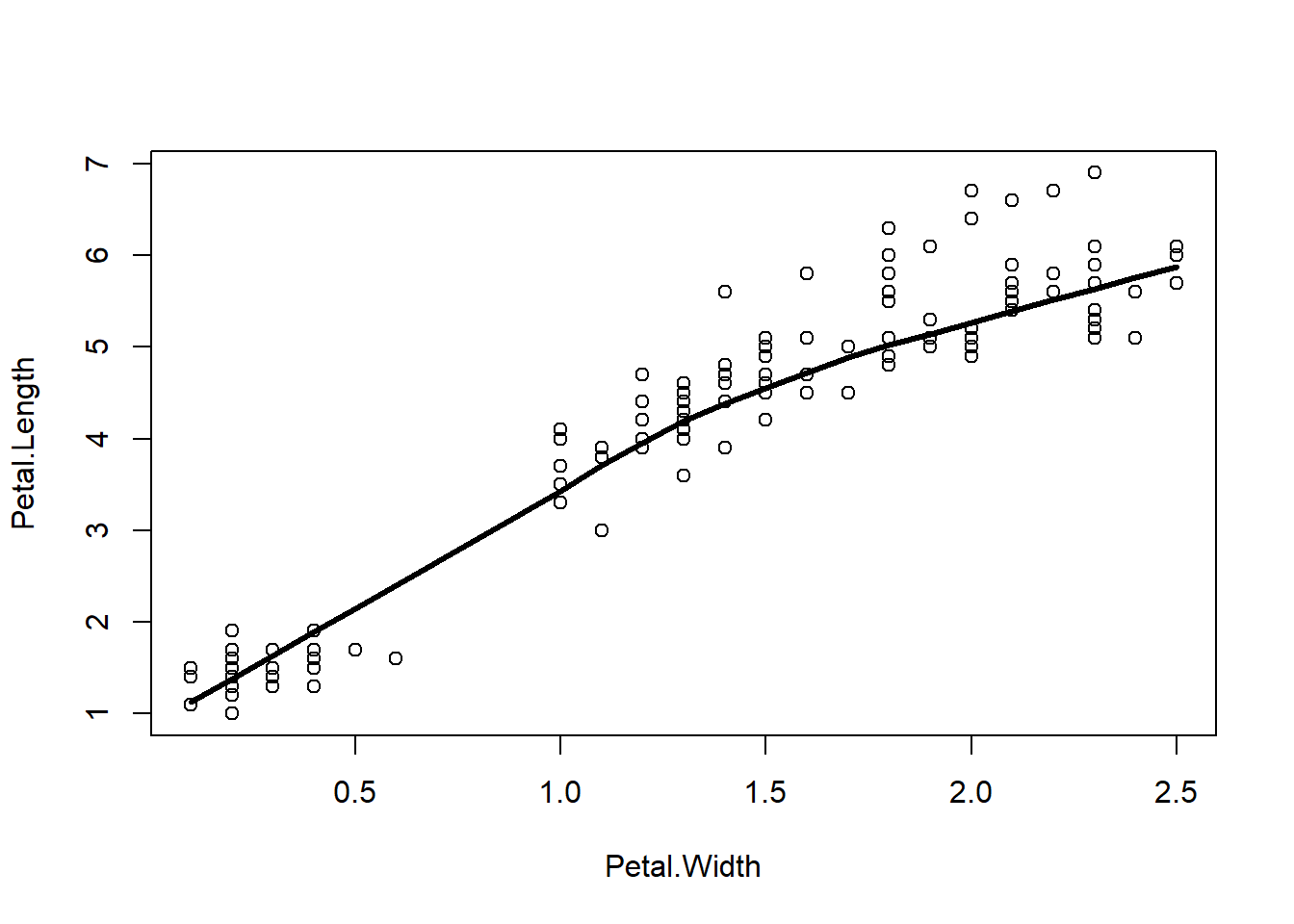# Using the comma for both functions, Petal length is on the x-axis
plot(iris$Petal.Length, iris$Petal.Width)
lines(lowess(iris$Petal.Length, iris$Petal.Width), lwd=3)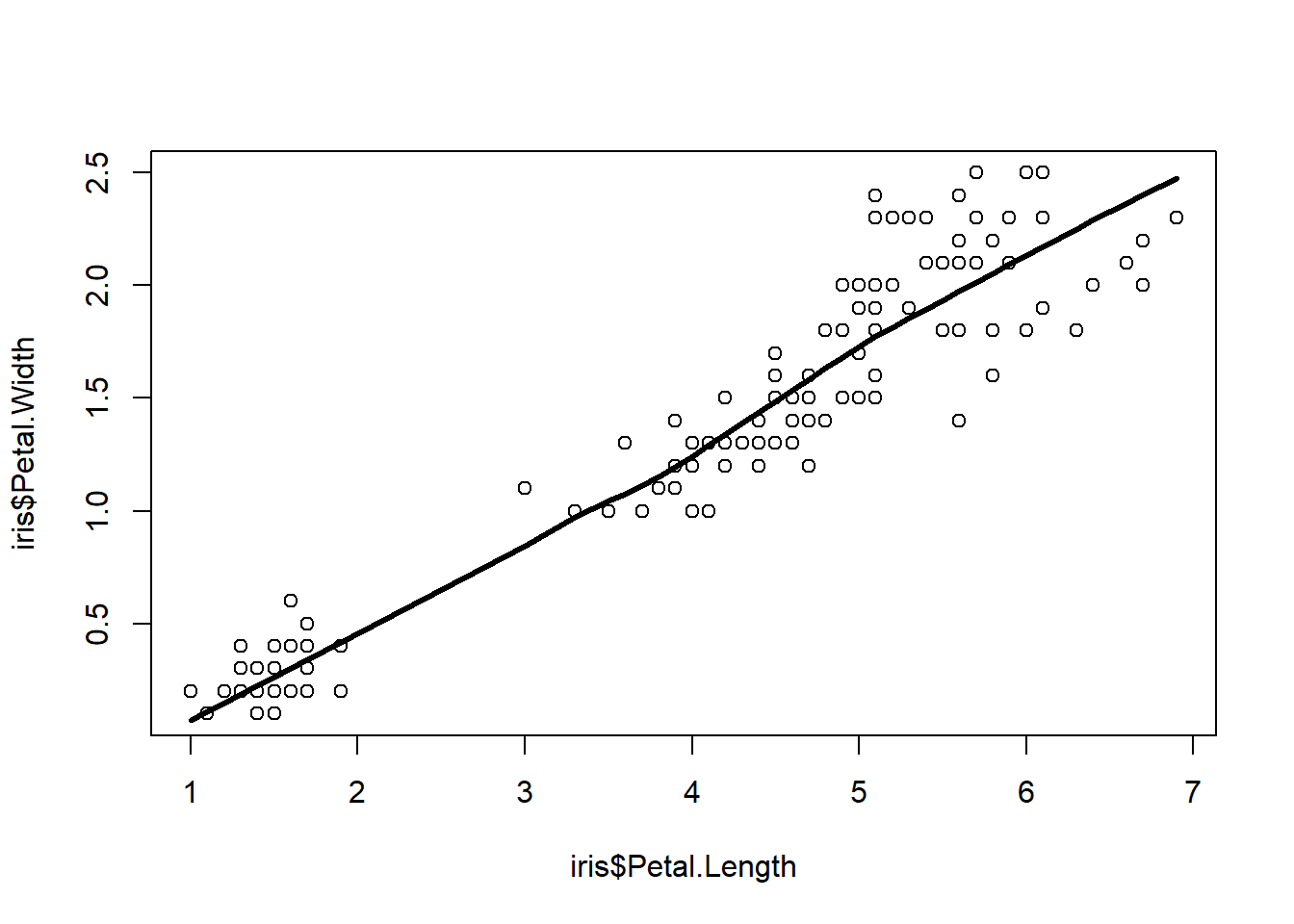# Mixing the ~ and the comma, trendline isn't show because it's outside of the plot range
plot(Petal.Length ~ Petal.Width, data=iris)
lines(lowess(iris$Petal.Length, iris$Petal.Width), lwd=3)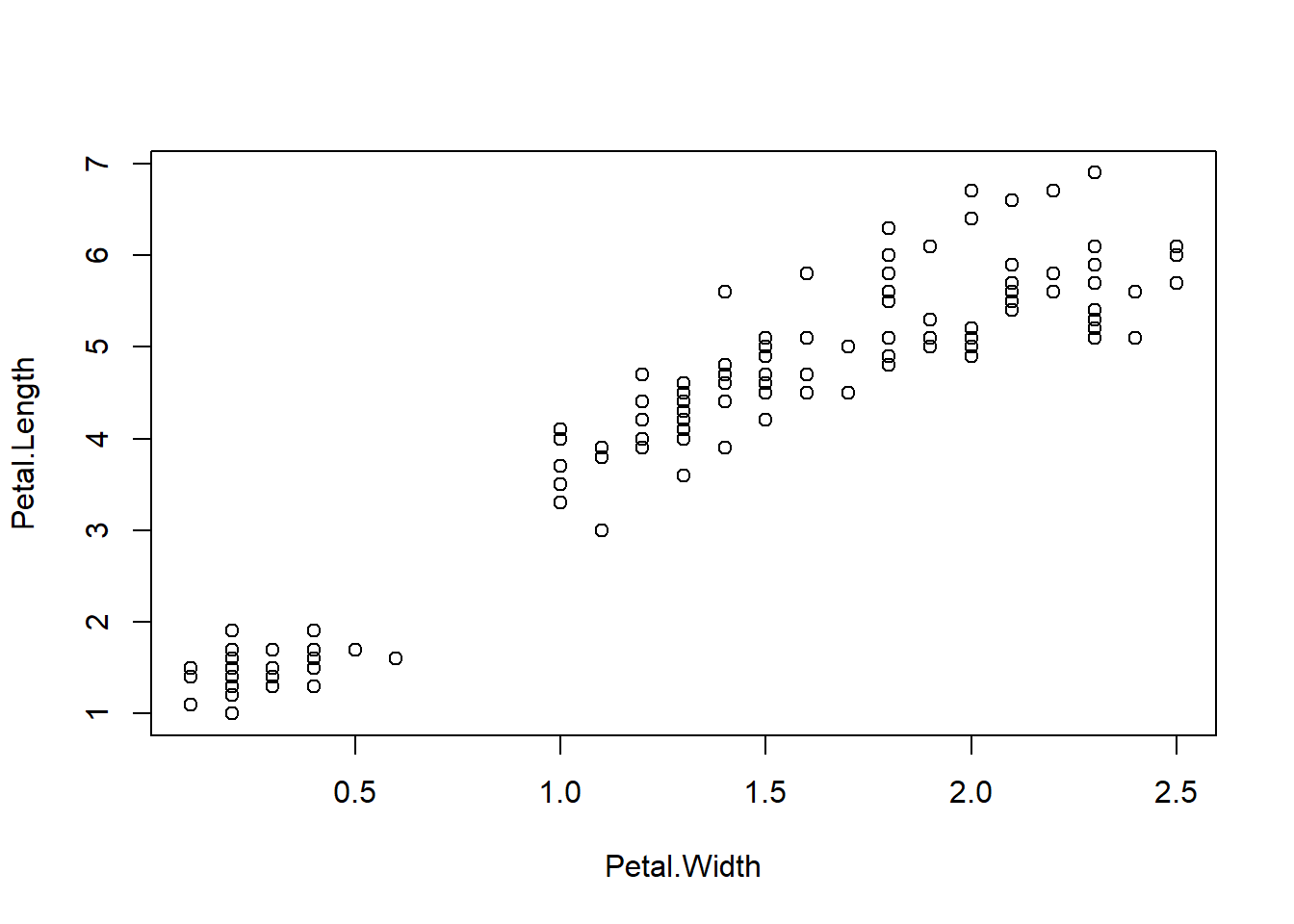# Try extending the plot range
plot(Petal.Length ~ Petal.Width, data=iris, xlim = c(0,10), ylim = c(0,10))
lines(lowess(iris$Petal.Length, iris$Petal.Width), lwd=3)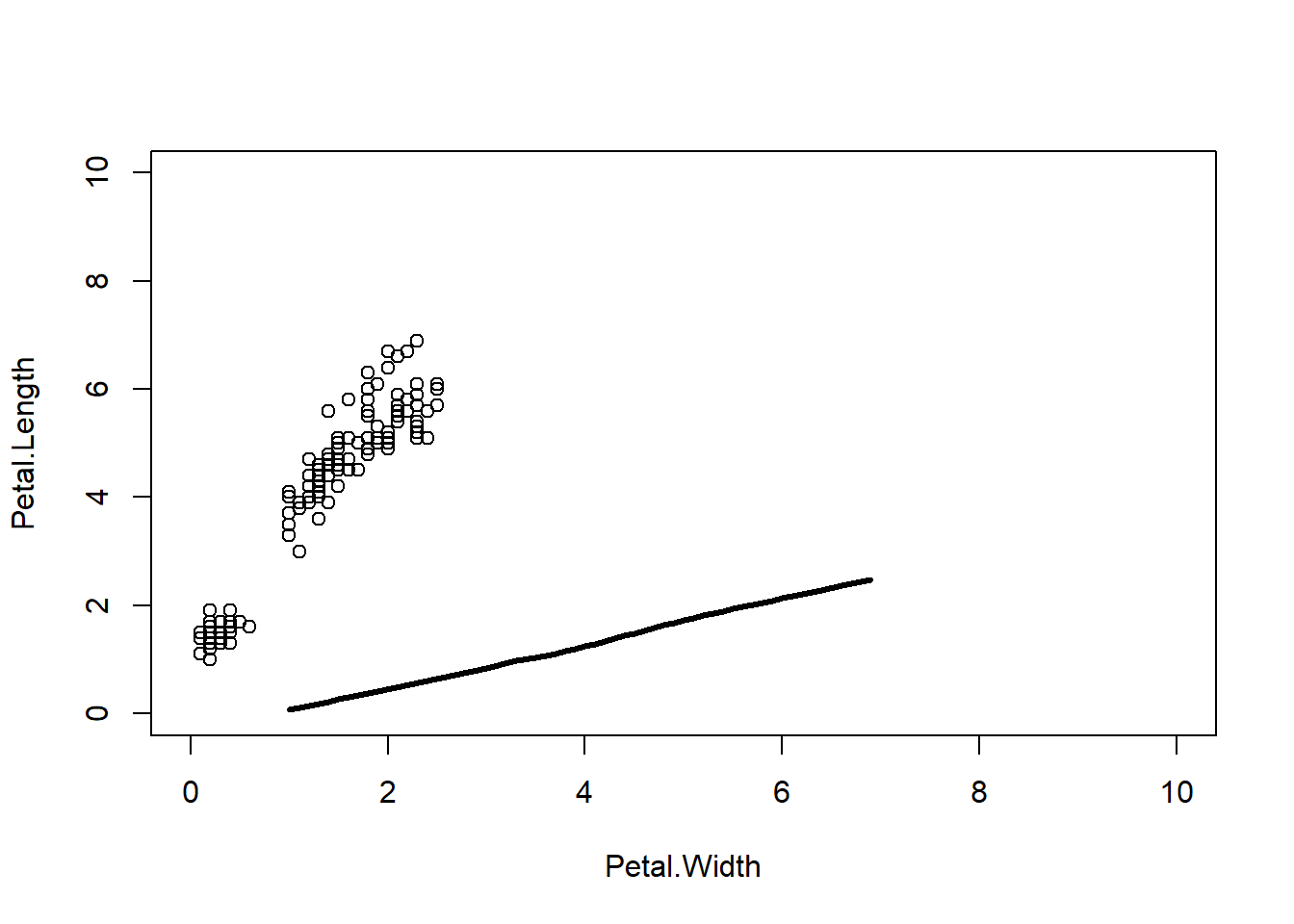### Other notes

• If one line of code gets very long, you can break it up by placing line breaks using the Enter key, one way to do this is to place a line break after the ending an argument with a comma ,:
data2=read.fwf('climate change data.txt',
widths=c(rep(6,3),7,10),
col.names=c('deltaT','sdev','proxy','T.M','latitude'))
• There were some more questions on subsetting data, take a look at the Module 3 Notes for some more examples on way to subset data frames.

• The c in the c() function stands for concatenate, which means “to link things together in a chain or series”. In R the c() function is used to group elements into a vector. Recall that are elements in a vector must be the same data type (e.g., numeric, character, logical).

• Press Tab to bring up the auto-complete menu, for instance after data\$ to bring up a menu with all of the column names in data.

• You can use a digital copy of your notes for the final, which can consist of R scripts and text files (Word docs, pdf, etc.).

• Note that the “Select all that apply” questions in the quizzes can only have one correct answer.